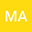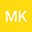Numerical and theoretical treatment based on the compact finite difference and Spectral collocation algorithms of the space fractional-order Fisher's equation
••Cairo Univeisty
Author ProfileBenha University
Author Profile## Abstract

This paper present an accurate numerical algorithm to solve the space fractional-order Fisher’s equation where the derivative operator is described in the Caputo derivative sense. In the presented discretization process, first we use the compact nite difference (CFD) to occur a semi-discrete in time derivative, and implement the Chebyshev spectral collocation method (CSCM) of the third-kind to discretize the spatial fractional derivative. The presented method converts the studied problem to be a system of algebraic equations which can be easily solved. To study the convergence and stability analysis, some theorems are given with their profs. A numerical simulation is given to test the accuracy and the applicability of our presented algorithm.

#### Peer review status:UNDER REVIEW

05 Mar 2020Submitted to Mathematical Methods in the Applied Sciences
07 Mar 2020Assigned to Editor
07 Mar 2020Submission Checks Completed
12 Mar 2020Reviewer(s) Assigned